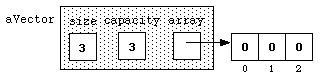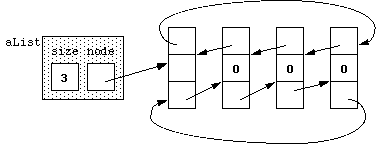# Lab 13: The `list` Class Template

## Introduction

We have seen that the `vector` container provided by the Standard Template Library (STL) can be used to store a sequence of values that are all of the same type. Another useful STL container for storing sequences of values is `list`. Except for `operator[]`, `at()`, `capacity()`, and `reserve()`, any of the operations that can be applied to a `vector` can also be applied to a `list`.

This lab exercise will explore the differences between these two containers — how they differ, under what circumstances should each be used. It consists of five experiments to time how long it takes to perform each of five different operations on each container.

1. Declare (i.e., create) an n-element `list` or `vector`.
2. Append a value to the end of a `list` or `vector` of length n.
3. Append n values to the end of an empty `list` or `vector`.
4. Prepend one value to the beginning of a `list` or `vector` of length n.
5. Prepend n values to the beginning of an empty `list` or `vector`.
In each case, the value n will be entered from the keyboard.

## Files

• `Timer.h` and `Timer.txt` implement the timer used in all of the experiments (there is no `Timer.cpp`).
• Each experiment has its own driver that runs the timing tests using the `Timer` class.
For all of these experiments, you will not have to change any of the code.

## Comparing `list` and `vector`

### Picturing a `vector`

Consider this declaration:

`vector<int> aVector(3);`
This defines `aVector` as an object that stores three `int` values, and it initializes each element to zero (the default `int` value). We might picture it asOne key property of a `vector` is that the elements of a vector are allocated in adjacent memory locations. That is, in the computer's memory, `aVector` is physically next to `aVector`, which is physically next to `aVector`, and so on.

### Picturing a `list`

`list<int> aList(3);`
This allocates a sequence of linked nodes, consisting of a head node and three additional nodes, linked together with pointers. We can picture this asA pointer is a special kind of variable that can store the address of an object. Each node in a `list` contains two pointers: one pointing to the next node in the sequence and the second pointing to the previous node, as pictured above.

Because these nodes are connected using pointers, they need not be in adjacent memory locations; they can be anywhere in a program's free store of memory. By following the pointers, we can get from `aList` to the the node at the head of the list without any data in it. This node is called the head node and is there to provide a starting node for the next and previous pointers. Using the next-node pointer of the header node we can get to the first node of the `list`; from its next-node pointer we can get to the second node of the `list`; and so on. We can go through the `list` in reverse order by following the previous-node pointers. A `list`'s nodes can be scattered throughout memory and the order of the values in the `list` is determined solely by the next-node and previous-node pointers of the nodes.

### Accessing Elements

Because the elements of a `vector` are stored in adjacent memory locations, the subscript operation can be performed efficiently for a `vector`. The expression `aVector[i]` to access the `i`-th element of `aVector` involves a simple arithmetic computation:

`aVector.array + (i * sizeof(int))`
By contrast, trying to access the i-th element of a `list` requires starting at the pointer stored in `node` and following it to the head node; then follow its next-node pointer to reach node #1; follow its next-node pointer to get to node #2; and so on until we reach node #i. This requires a loop and doing a computation for each pointer we follow, which is obviously more time consuming that the `vector`'s simple math computation.

## The `Timer` Class

The `Timer` class stored in the file `Timer.h` can be used to measure the elapsed time in each experiment below. It computes time in seconds like a stopwatch and provides the following operations:

Statement Meaning
` Timer aTimer;`  Constructs `aTimer` as a `Timer` object.
` aTimer.start();`  Starts `aTimer` running.
` aTimer.stop();`  Stops `aTimer`.
` aTimer.reset();`  Resets `aTimer` to zero.
` cout << aTimer;`  Displays the elapsed time recorded by`aTimer`.
` aTimer.getSeconds()`  Returns the current value of `aTimer`.

The `Timer` class is built using the `clock()` function from the standard C library `ctime`. This means that it is only as accurate as this `clock()` function.

Most of the operations that we test in this exercise are very fast — so fast that the program probably will not notice them running at all because the timer cannot measure fractional seconds very well. So, instead of timing just one run of a test, the programs run each test many, many times — 1000 times, by default — and then computes the average time. (Note: you can change the number 1000 by changing the value of the constant `ITERATIONS` if the tests take extraordinarily long on your computer.)

## The Drivers

The driver for each experiment does the following:

1. Prompt for and read a value for `n`, which is the size of the container in the first two experiments and the number of elements added to containers in the last three.
2. Run the current test on a `list` using `n`.
3. Run the current test on a `vector` using `n`.
4. Display the times for a `list` and for a `vector`.

Regardless of which container is being tested and the test being conducted, the tests themselves have the same structure:

1. Declare and start a timer.
2. Repeat the following `ITERATIONS` times:
1. Declare and construct the container.
2. Perform the test.
3. Stop the timer.
4. Return the total number of seconds divided by the number of iterations.
The "Perform the test" step is the only thing that changes program to program in testing the different operations.

## The Experiments

For each experiment, execute the program on sets of values ranging in size from 100 through 2000 in increments of 100. (Your instructor may have a different range of values to use, depending on the computer and compiler you use.)

Record your results and enter them into a spreadsheet. Then graph the results: mark off the values for `n` on the horizontal axis and the time it takes to do the operation on the vertical axis. Use different colors (or symbols) for the points, one for the `list` results and another for the `vector` results.